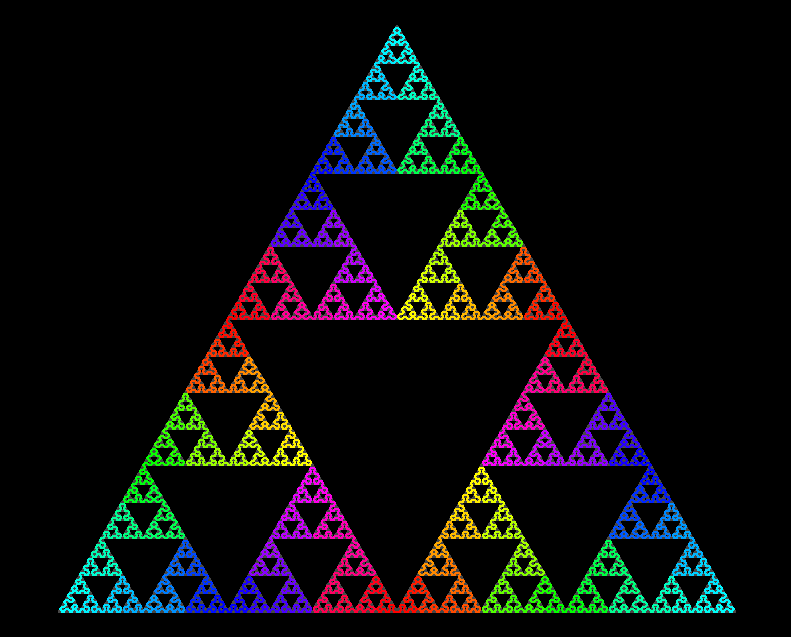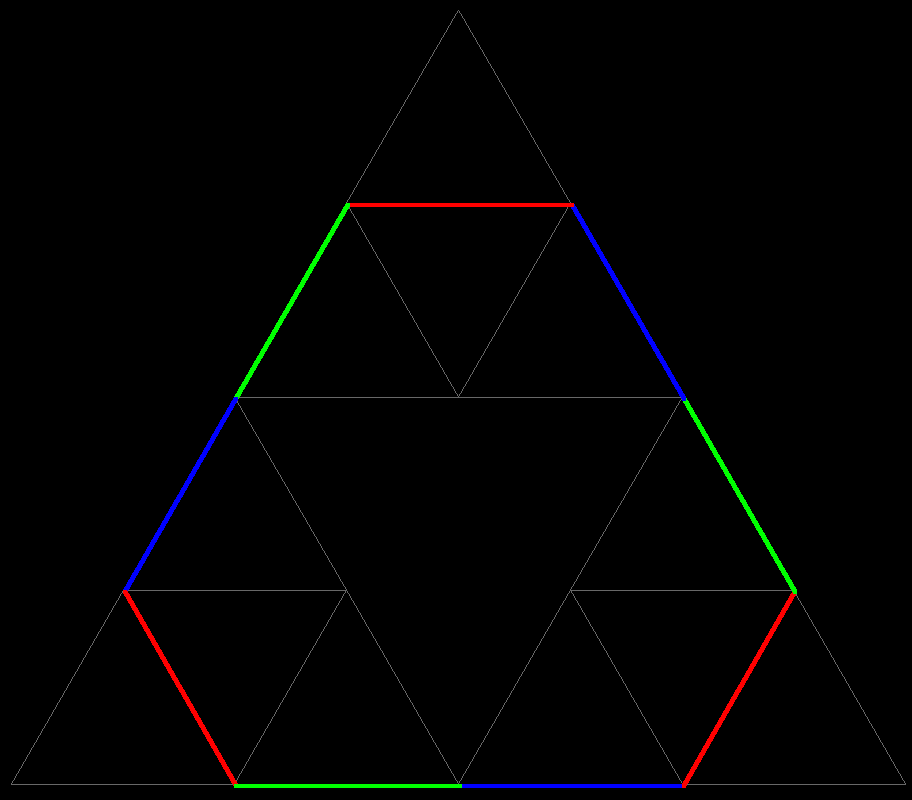# Sierpinski TriangleThe Sierpinski Triangle is usually described just as a set: Remove from the initial triangle its "middle", namely the open triangle whose vertices are the edge midpoints of the initial triangle. (open means: only the interior of the middle triangle is removed, not its edges.) Of the remaining 3 triangles remove again their middles. Of the remaining 9 triangles remove again their middles - and so on. Observe that no point on an edge of a removed triangle is removed in a later step, all these edges belong to the final set.The Sierpinski Triangle is more than just a set. It is the image of a continuous curve! This sequence shows the first few approximations by polygons. These polygons converge to a continuous limit curve whose image is the Sierpinski Triangle.

Sierpinski_Curve.pdf